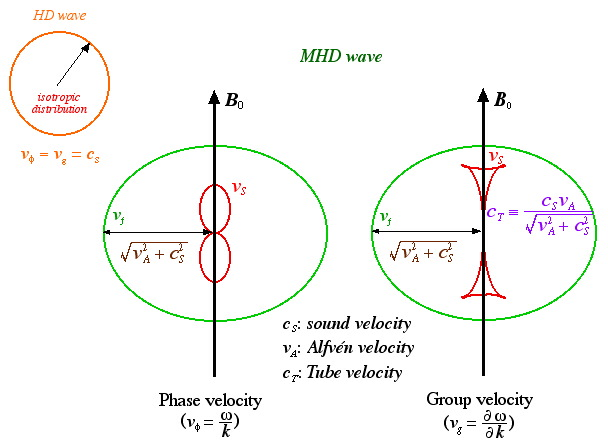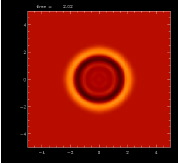## MHD Waves

Compared to hydrodynamic (HD) waves in a nonmagnetized fluid, magnetohydrodynamic (MHD) waves in a magnetized fluid show complicated behavior. Here we present the results of 2-dimensional MHD numerical simulations and explain the basic properties of MHD waves.

### Introduction

First of all, we show the dispersion-relation diagram of an HD wave (sound wave) and two MHD waves (fast-mode wave, slow-mode wave). Phase velocity is the propagation speed of a single-mode wave while group velocity is the propagation speed of a group of single-mode waves.Next we describe the basic setting of the simulations.### Simulation Results

In the following we show wave propagation in a non-magnetized fluid (HydroDynamic case), highly magnetized fluid (Low beta case), and weakly magnetized fluid (High beta case). Click the picture for details.

 HD case(Nonmagnetized case) Low beta case (beta = 0.5) (Strong magnetic field) High beta case (beta = 2.0) (Weak magnetic field)